Combining DataFrames with Pandas

• Last Updated : 11 Dec, 2020

Pandas DataFrame consists of three principal components, the data, rows, and columns. To combine these DataFrames, pandas provides multiple functions like concat() and append().

Method #1: Using concat() method

Initially, creating two datasets and converting them into dataframes.

Python3

 # import required moduleimport pandas as pd  # making  a datasetdata1 = {    'Serial_No.': ['1', '2', '3', '4', '5'],    'First': ['F0', 'F1', 'F2', 'F3', 'F4'],    'Second': ['S0', 'S1', 'S2', 'S3', 'S4'],}  # creating a dataframedf1 = pd.DataFrame(data1, columns=['Serial_No.',                                    'First',                                    'Second'])  # display dataframedf1    # making  a datasetdata2 = {    'Serial_No.': ['6', '7', '8', '9', '10'],    'First': ['F10', 'F11', 'F12', 'F13', 'F14'],    'Second': ['S10', 'S11', 'S12', 'S13', 'S14'],}  # creating  a datasetdf2 = pd.DataFrame(data2, columns=['Serial_No.',                                    'First',                                    'Second'])  # display datasetdf2

Output: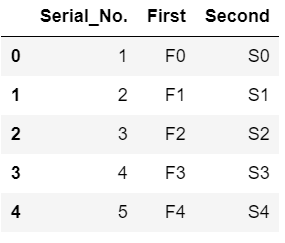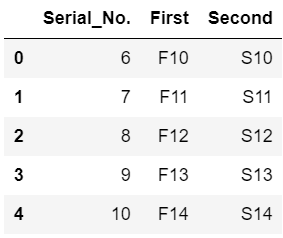Now, concatenating the two dataframes, we will use concat() to combine two dataframes. If ignore_index = True the index of df will be in a continuous order.

Python3

 # combining the two dataframesdf = pd.concat([df1, df2], ignore_index=True)  # display combined dataframesdf

Output: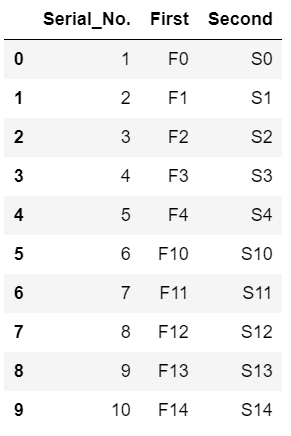Using keys we can specify the labels of the dataframes.

Python3

 # we can also separate 2 datasets using keysframes = [df1, df2]df_keys = pd.concat(frames, keys=['x', 'y'])  # display dataframedf_keys

Output: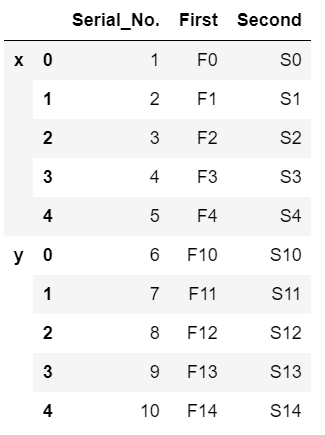Method #2: Using append() method

Initially, creating two datasets and converting them into dataframes.

Python3

 # import required moduleimport pandas as pd  # making  a datasetdata1 = {    'Serial_No.': ['1', '2', '3', '4', '5'],    'First': ['F0', 'F1', 'F2', 'F3', 'F4'],    'Second': ['S0', 'S1', 'S2', 'S3', 'S4'],}  # creating a dataframedf1 = pd.DataFrame(data1, columns=['Serial_No.',                                    'First',                                    'Second'])  # display dataframedf1    # making  a datasetdata2 = {    'Serial_No.': ['6', '7', '8', '9', '10'],    'First': ['F10', 'F11', 'F12', 'F13', 'F14'],    'Second': ['S10', 'S11', 'S12', 'S13', 'S14'],}  # creating  a datasetdf2 = pd.DataFrame(data2, columns=['Serial_No.',                                    'First',                                    'Second'])  # display datasetdf2

Output:The dataframe.append() method performs the operation of combining two dataframes similar to that of the contcat() method.

Python3

 # combining dataframesresult = df1.append(df2, sort=False, ignore_index=True)  # display combined dataframeresult

Output: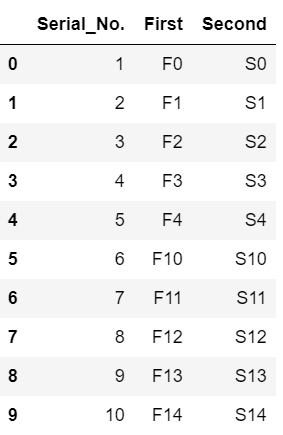Attention geek! Strengthen your foundations with the Python Programming Foundation Course and learn the basics.

To begin with, your interview preparations Enhance your Data Structures concepts with the Python DS Course. And to begin with your Machine Learning Journey, join the Machine Learning - Basic Level Course

My Personal Notes arrow_drop_up